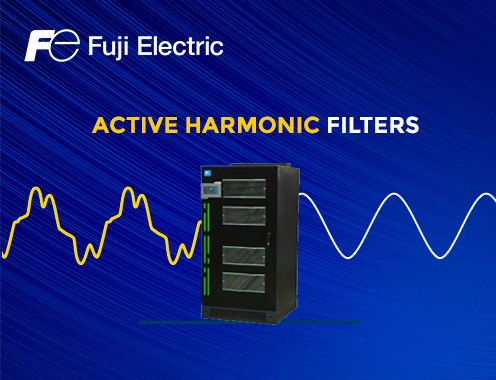# Active harmonics filter for power quality improvementWhat are harmonics and the effect?

Harmonics are higher frequency waveforms superimposed onto the fundamental frequency, that is the frequency of the circuit, and which are sufficient to distort its wave shape. In an electric power system, a harmonic of a voltage or current waveform is a sinusoidal wave whose frequency is an integer multiple of the fundamental frequency.

Harmonics have only been around in sufficient quantities over the last few decades since the introduction of electronic drives for motors, fans and pumps, power supply switching circuits such as rectifiers, power converters and thyristor power controllers as well as most non-linear electronic phase-controlled loads and high frequency (energy saving) fluorescent lights. This is due to the fact that the controlled current drawn by the load does not faithfully follow the sinusoidal supply waveforms as in the case of rectifiers or power semiconductor switching circuits. Harmonic frequencies are the frequent cause of power quality problems and can result in an increased equipment and conductor heating, misfiring in variable speed drives, and torque pulsations in motors and generators.

Harmonic Filters

Harmonics filters go a long way in making electrical usage more efficient and effective. Passive harmonics filters are the more common devices used in a variety of capacities and voltages. These filters utilize components like inductors, capacitors and resistors. Passive filters generally filter noise on a single variable speed drive. They eliminate harmonics before the electrical current reaches the equipment.

Active harmonics filters can work with many variable speed drives and actively reduce noise by constantly monitoring electricity and injecting currents to mitigate harmonics. Active filters are ideal for a system with many non-linear loads.

Harmonics & Energy Loss – An Example

Increase current:

• 415Vac, 90Amp, 0.85PF
• VFD with DC Link Reactor, generate 40% Harmonics

𝐼𝑖𝑛 = (𝐾𝑊×1000) / (415×1.73×0.92)

83A = (55×1000) / (415×1.73×0.92)

𝐼RMS = √(1²+THiD²) × 𝐼𝑓

89.7𝐴= √(1²+0.4²) × 83                   (Without VFD losses)

Displacement power factor close to unity (Cosø 0.98); True Power factor > 0.9

Total Harmonic Distortion

pẝTRUE ~ 1 / √(1+(THiD/100)²)

• For THID ~45% True Power factor will be 0.92
• For THID ~40% True Power factor will be 0.93
• For THID ~5% True Power factor will be ~ 0.98

Better energy efficiency can be achieved by using the correct harmonics solution with VFD.